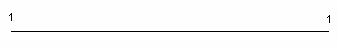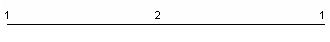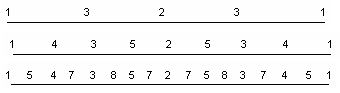favorite We need a little bit of your help to keep things running, click on this banner to learn more
Problems

# Sequence

Consider the segment, at the end of which the ones are written. Then till infinity we shall do the next procedure: for each segment with ends a and b (inside which the numbers are absent) we shall write in the middle the number a + b. So from the starting segmentwe getThen we get the segmentand so on till infinity. How many times the positive integer n will be written on a segment?

#### Input

One positive integer n (n`1013`).

#### Output

The number of times the number n appears on a segment.

Time limit 1 second
Memory limit 128 MiB
Input example #1
```4
```
Output example #1
```2
```
Source III International Summer School Programming in Sevastopol 2012